Linear Systems of Conics

Conics.
A "conic curve" (or a "conic section," or just a "conic") in the two-dimensional plane is the solution set of an equation of the form:
Ax2 + By2 + Cxy + Dx + Ey + F = 0
for real constant coefficients A, B, C, D, E, F, which are not all zero. There are different types of conic curves in the real plane. The following chart gives a representative for each type of quadratic equation, and a name for the corresponding conics. For our purposes, they are divided into five cases:
 Real Nondegenerate Imaginary Nondegenerate Ellipse x2+y2-1=0 Imaginary Ellipse x2+y2+1=0 Hyperbola x2-y2-1=0 Parabola x2-y=0
 Real Degenerate Imaginary Degenerate Intersecting Pair of Lines x2-y2=0 Imaginary Pair of Lines, Intersecting at a Real Point x2+y2=0 Parallel Lines x2-1=0 Imaginary Parallel Lines x2+1=0 Pair of Lines, one at Infinity x=0
 Square Degenerate Coincident Lines x2=0 Coincident Lines at Infinity 1=0
If you know the coefficients of a quadratic equation, you can find its type in the above chart, by using the table from a Geometry Center web page. We can abbreviate an equation as E1(x,y) = 0, and multiplying all the coefficients of E1 by the same nonzero constant, c, gives
(c . E1)(x,y) = cAx2 + cBy2 + cCxy + cDx + cEy + cF.
Then E1(x,y) = 0 if and only if (c . E1)(x,y) = 0, so E1 = 0 and c . E1 = 0 have the same solution set, and they define the same conic curve.

Linear systems.
A "linear system of conics" (also called a "pencil of conics") is the set of linear combinations of polynomials E1 and E2:
t1 . E1 + t2 . E2.
We assume E1 and E2 are linearly independent: one is not a constant multiple of the other. Plugging specific numbers into these coefficients, (t1,t2), gives a polynomial in (x,y), so each ordered pair (t1,t2) corresponds to a conic curve (t1 . E1 + t2 . E2)(x,y) = 0. Multiplying both t1 and t2 by the same nonzero constant c results in the same curve:
((c . t1) . E1 + (c . t2) . E2)(x,y) = c . (t1 . E1 + t2 . E2)(x,y) = 0.
So, a linear system is really just a one-parameter family of different conic curves, and the non-zero pairs (t1,t2) could be considered as homogeneous coordinates [t1 : t2] for a projective line. (See my Steiner surfaces page for some details on homogeneous coordinates.)

• Theorem: In a real linear system of conics, there is at least one conic which contains a real line (so that conic is either "real degenerate" or "square degenerate").
• Theorem: If there is one nondegenerate conic in a linear system, then there are at most three degenerate conics. However, there exist linear systems where all the conics are degenerate.
Intersections.
A point (x,y) is on both conics if E1(x,y) = 0 and E2(x,y) = 0. Any point on this intersection will also be a point on every conic in the linear system: for any t1, t2,
(t1 . E1 + t2 . E2)(x,y) = t1 . 0 + t2 . 0 = 0.
In fact, if any two conics in a linear system meet at a point, then that point is on every member of the linear system. Such points of intersection are called base points. If two conics in a linear system are disjoint, then every pair of conics in the linear system will also be disjoint, and there are no base points.

• Theorem: If a real linear system of conics has finitely many base points, then there can be 0, 1, 2, 3, or 4 of them, but no more than four. However, there exist linear systems with infinitely many base points.
• Theorem: Every point in the plane which is not a base point lies on exactly one conic in the linear system.
The Classification
The quadratic equation of a conic could also be written as a homogeneous expression in three variables, Ax2 + By2 + Cxy + Dxz + Eyz + Fz2 = 0, which gives the original x,y equation when z = 1. This corresponds to the geometric definition of a conic section as the intersection of a cone (defined by the homogeneous equation in xyz-space) with a plane (defined by z = 1).

The following examples list all the types of linear systems of conics, up to "real projective equivalence" (real linear transformations of the homogeneous coordinates [x : y : z]). It turns out there are only finitely many types of linear systems; roughly speaking, they can be categorized by the number of base points and degenerate conics, but there are the usual issues of "multiplicity," and whether the base points have real coordinates.

The numbering system for types I, Ia, ..., V follows H. Levy's book. The Roman numerals I, ..., VIII represent eight complex projective equivalence classes, some of which are further subdivided into real projective equivalence classes, for a total of 13 types.

Click on the picture to see an animation.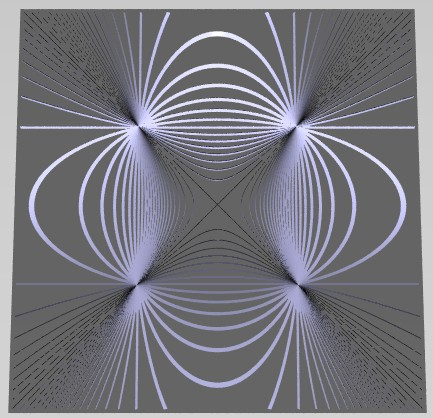Type I t1 . (x2-y2) + t2 . (x2-1). These conics meet at four real base points. There are three degenerate conics: [t1:t2]=[1:0] The intersecting lines x2-y2=0, [t1:t2]=[0:1] The vertical parallel lines x2-1=0, [t1:t2]=[-1:1] The horizontal parallel lines y2-1=0. To see how I rendered the conic sections in the picture to the left, Click Here to see a "side view," showing the cones in xyz-space, whose intersection with the plane z=1 (the top of the box) is shown in the two-dimensional figure.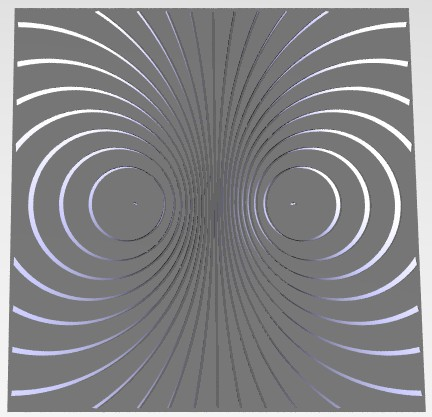Type Ia t1 . (x2+y2+1) + t2 . (x). The type Ia linear system has no real base points. In this representative, most of the conics you see in the picture are circles, but there are also "imaginary ellipses" with no visible points: for some values of [t1:t2], the polynomial equation (t1 . E1 + t2 . E2)(x,y) = 0 has no real solutions. There are three degenerate conics: [t1:t2]=[0:1] The vertical line x=0, [t1:t2]=[1:2] The point (-1,0) is the only real solution of x2+2x+1+y2=0, [t1:t2]=[1:-2] The point (1,0) is the only real solution of x2-2x+1+y2=0. In complex homogeneous coordinates, the linear system t1 . (x2+y2+z2) + t2 . (xz) = 0 has four base points: [0:i:1], [0:-i:1], [1:i:0], [1:-i:0].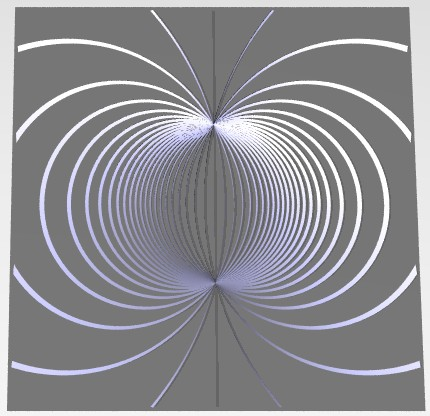Type Ib t1 . (x2+y2-1) + t2 . (x). The type Ib linear system has two real base points. Since all the conics in the system must meet both real base points, there are no "imaginary ellipses." There is one degenerate conic: [t1:t2]=[0:1] The vertical line x=0. In the complex linear system, where x, y, t1, and t2 are allowed to be complex, there are two more degenerate conics, at [t1:t2]=[1:2i] and [1:-2i]. In complex homogeneous coordinates, there are two more base points, [1:i:0], [1:-i:0].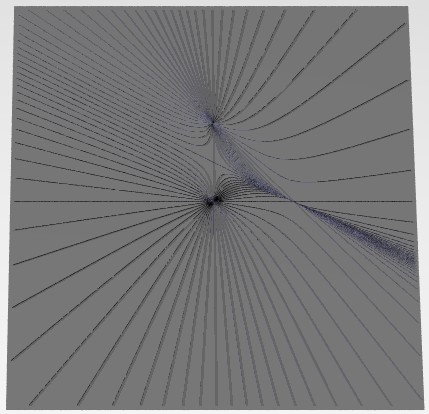Type II t1 . y(x+y-1) + t2 . x(x+2y-1). The type II linear system has three real base points, and at one of these points, all the nondegenerate conics have a common tangent line. There are two degenerate conics: [t1:t2]=[1:0] The pair of lines y(x+y-1)=0. [t1:t2]=[0:1] The pair of lines x(x+2y-1)=0.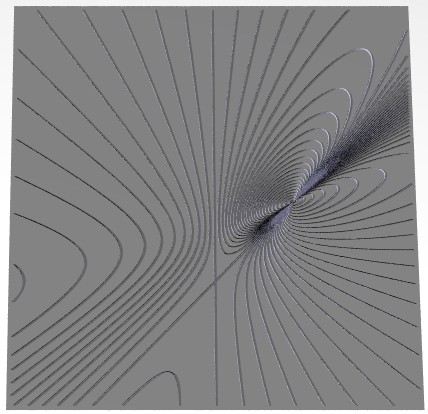Type IIa t1 . (y2+(x-y-1)2) + t2 . x(x-y-1). The type IIa linear system has one real base point, at which all the nondegenerate conics have a common tangent line. There are two degenerate conics: [t1:t2]=[1:0] The pair of imaginary lines y2+(x-y-1)2=0, meeting at the real base point (1,0). [t1:t2]=[0:1] The pair of lines x(x-y-1)=0. In complex coordinates, there are two more base points, (0,(-1+i)/2), (0,(-1-i)/2).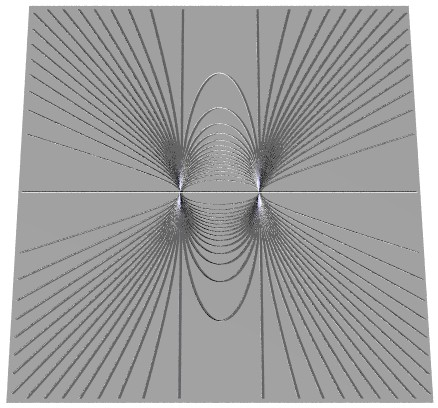Type III t1 . (x2-1/4) + t2 . y2. The type III linear system has two real base points. At each of the two base points, all the nondegenerate conics have a common tangent line. There are two degenerate conics: [t1:t2]=[1:0] The vertical parallel lines x2-1/4=0, [t1:t2]=[0:1] The horizontal coincident lines y2=0.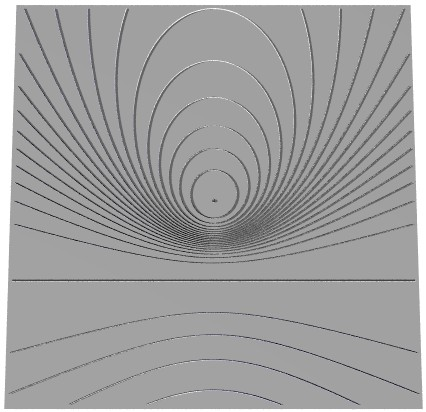Type IIIa t1 . (x2+y2) + t2 . (y+1)2. The type IIIa linear system has no real base points. Like Type Ia, there are some imaginary ellipses. There are two degenerate conics: [t1:t2]=[1:0] The point (0,0) is the only real solution of x2+y2=0, [t1:t2]=[0:1] The horizontal coincident lines (y+1)2=0. In complex coordinates, there are two base points, (i,-1), (-i,-1).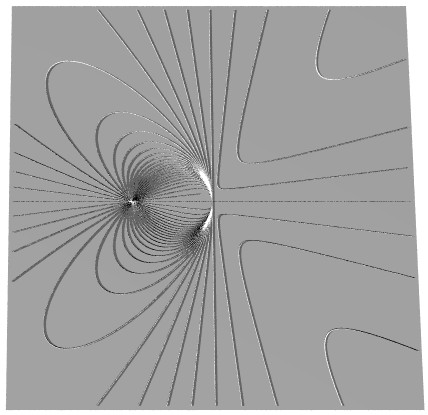Type IV t1 . (x2+x+y2) + t2 . (xy). The type IV linear system has two real base points. At one of the base points, any two conics meet each other transversely, and at the other, they meet at an intersection with multiplicity 3. There is only one degenerate conic: [t1:t2]=[0:1] The pair of lines xy=0.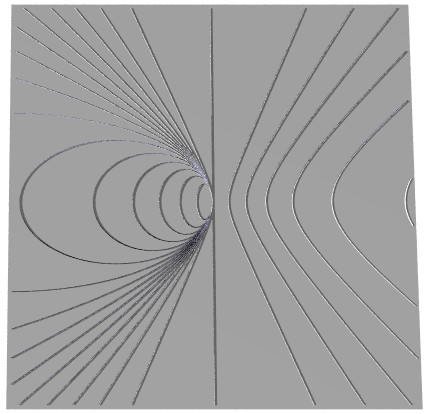Type V t1 . (x+y2) + t2 . (x2). The type V linear system has one real base point, at which any two conics meet each other at an intersection with multiplicity 4. There is only one degenerate conic: [t1:t2]=[0:1] The vertical coincident lines x2=0.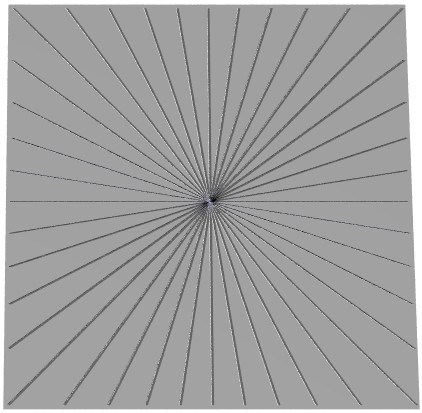Type VI t1 . (x2-y2) + t2 . (2xy). The type VI linear system has only real degenerate conics, which are pairs of lines that meet at the one real base point. In the complex linear system, where x, y, t1, and t2 are allowed to be complex, there are two square degenerate conics, at [t1:t2]=[1:i] and [1:-i].Type VIa t1 . (x2+y2) + t2 . (2xy). The type VIa linear system has real and imaginary degenerate conics, which are pairs of lines that meet at the one real base point. There are also two square degenerate conics, at [t1:t2]=[1:1] and [1:-1]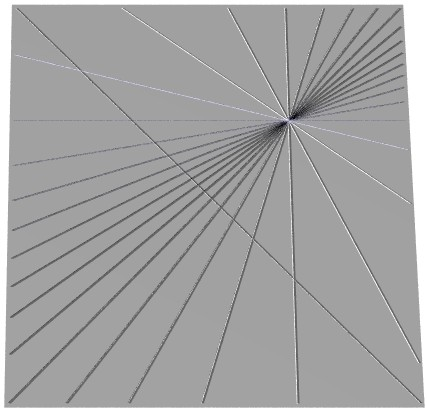Type VII t1 . (x2-y2) + t2 . (x+y)(y-1). The type VII linear system has only real degenerate conics, which are pairs of lines, one of which is a line of base points. There is also one real base point not on that line. There are no square degenerate conics.Type VIII t1 . (x2) + t2 . (xy). The type VIII linear system has base points which form exactly one line. There are real degenerate conics, and one square degenerate conic at [t1:t2]=[1:0]. This case is related to the previous one, by moving the isolated base point so that it is on the line of base points.